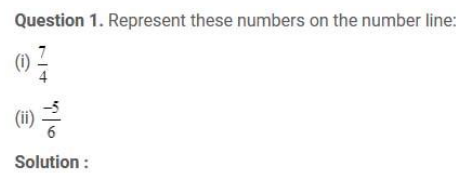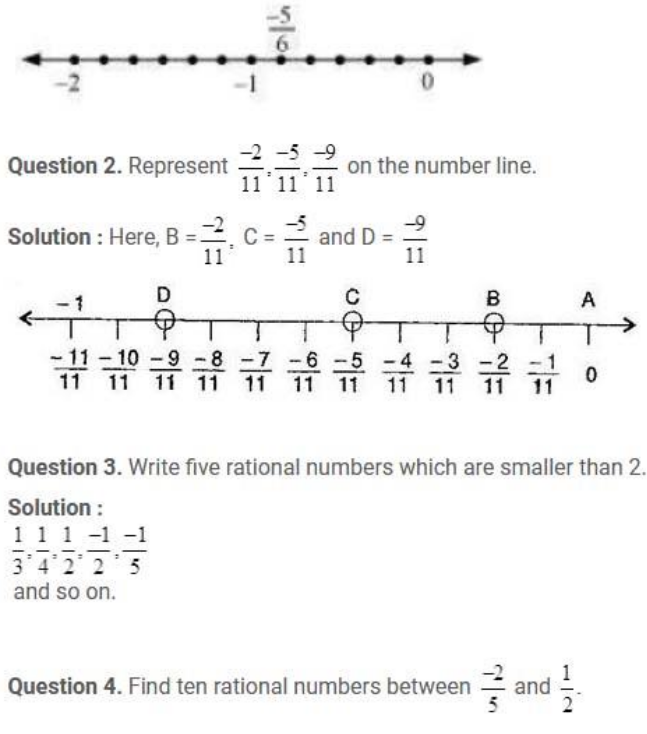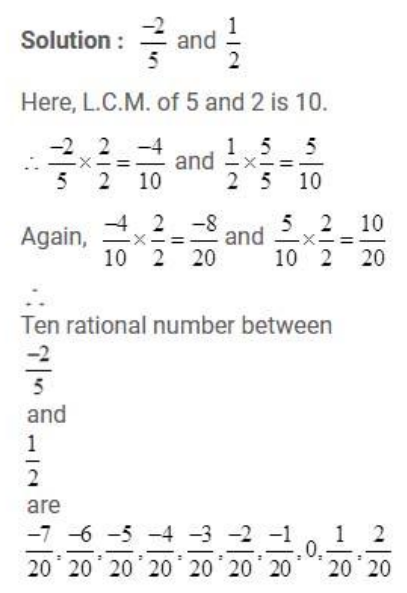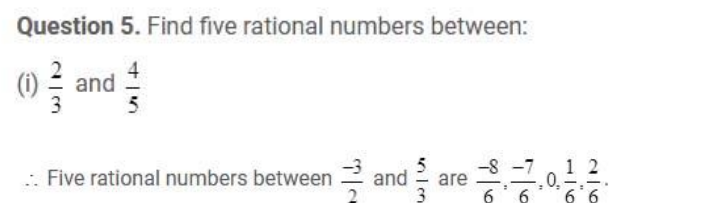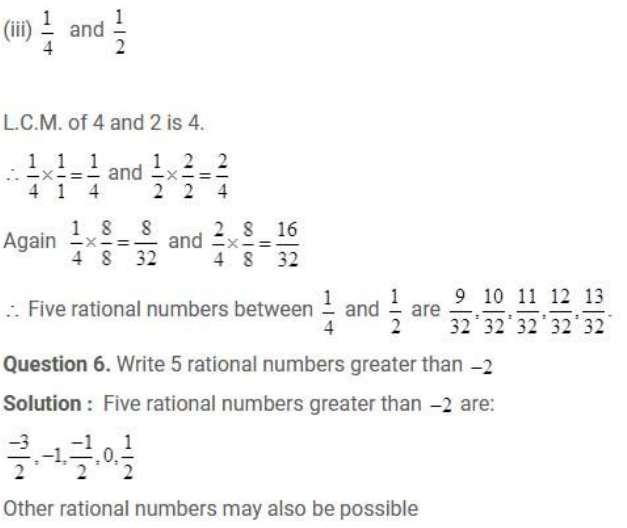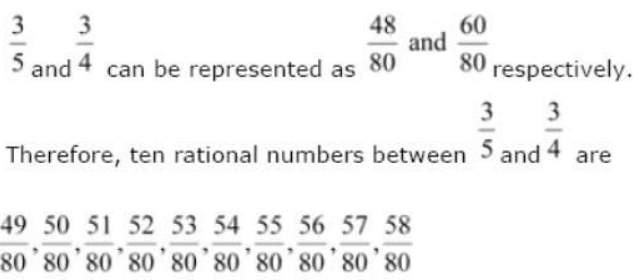# NCERT Solution for class 8 maths chapter 1-Rational Numbers

NCERT solutions for class 8 maths chapter 1is prepared by academic team of Physics Wallah. we have prepared solutions for all exercise of chapter-1. Given below is step by step solutions of all questions given in NCERT textbook for chapter-1 . Read chapter-1 theory make sure you have gone through the theory part of chapter-1 from NCERT textbook and you have learned the formula of the given chapter. Physics Wallah prepared a detail notes and additional questions for class 8 maths with short notes of all maths formula of class 8 maths . do read these contents before moving to solve the exercise of NCERT chapter 1

### NCERT Solutions for Class 8 Maths Exercise 1.1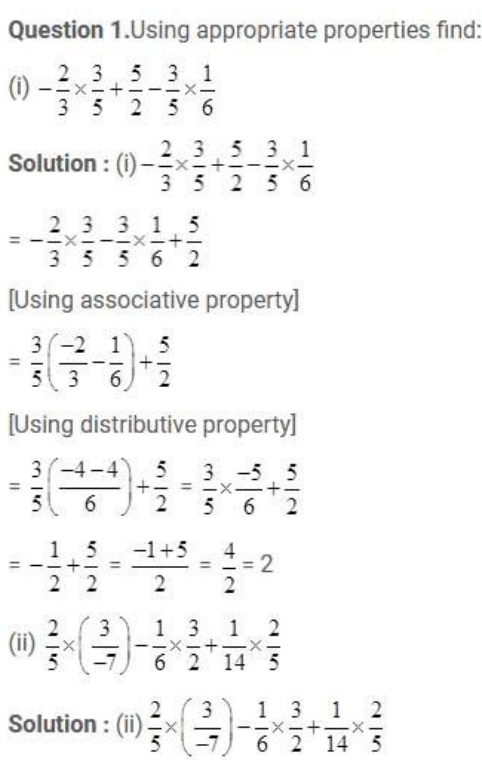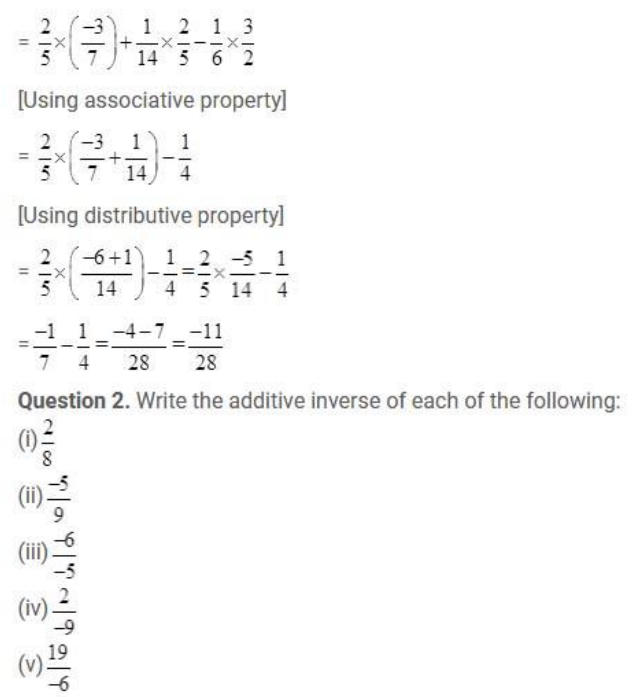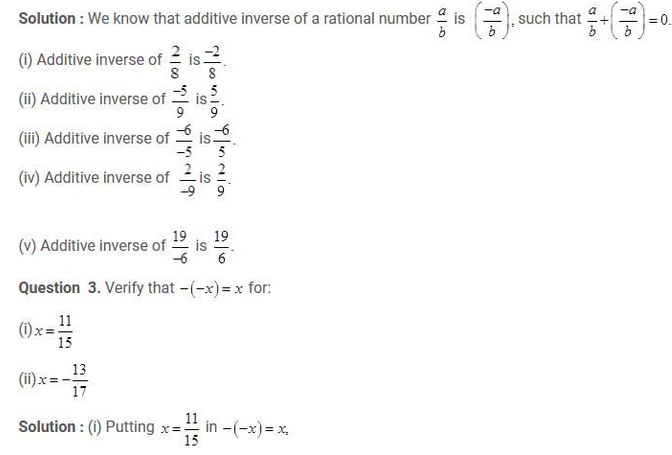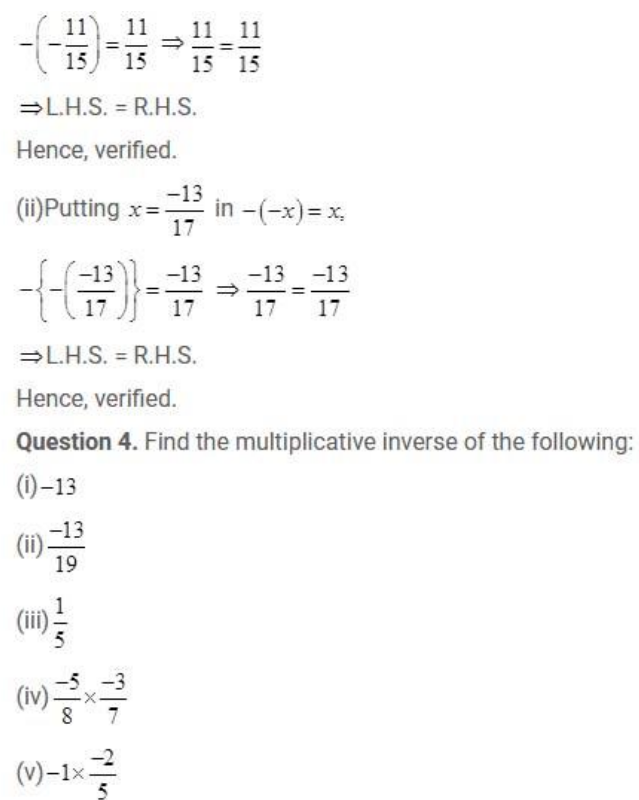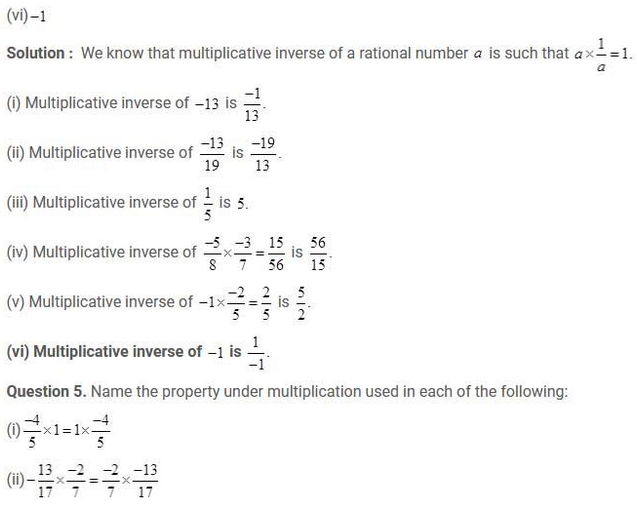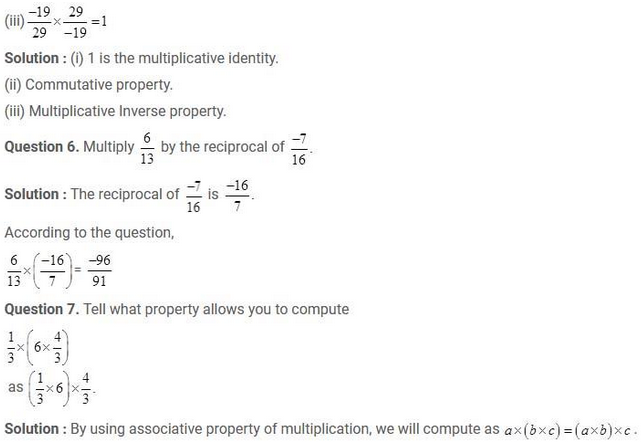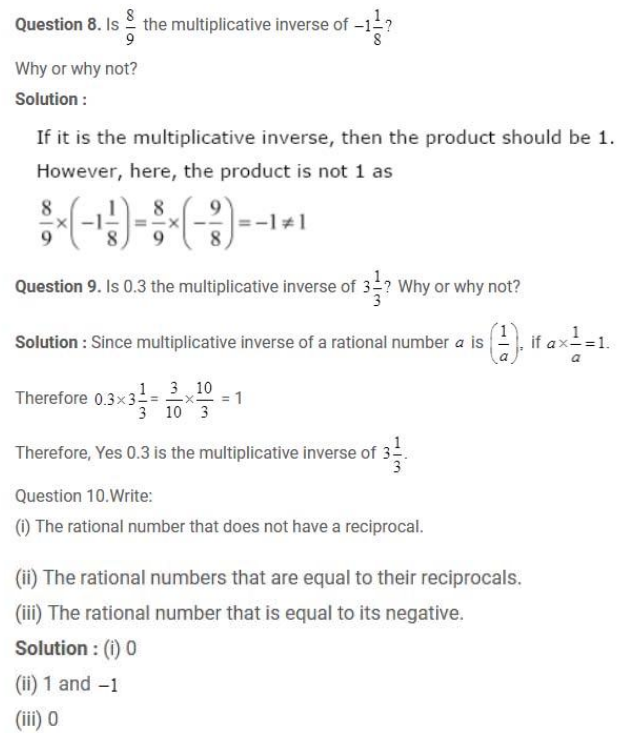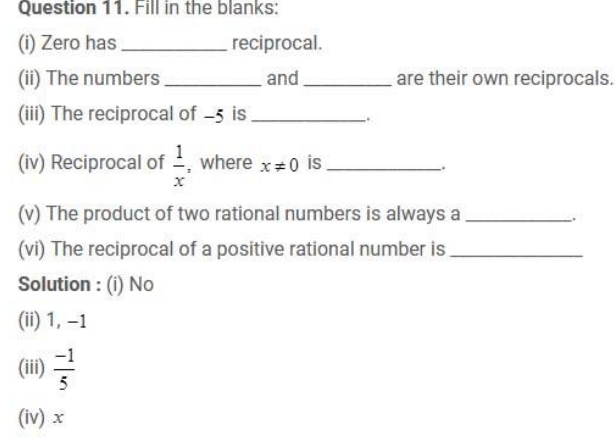(v) Rational Number

(vi) Positive

### NCERT Solutions for Class 8 Maths Exercise 1.2